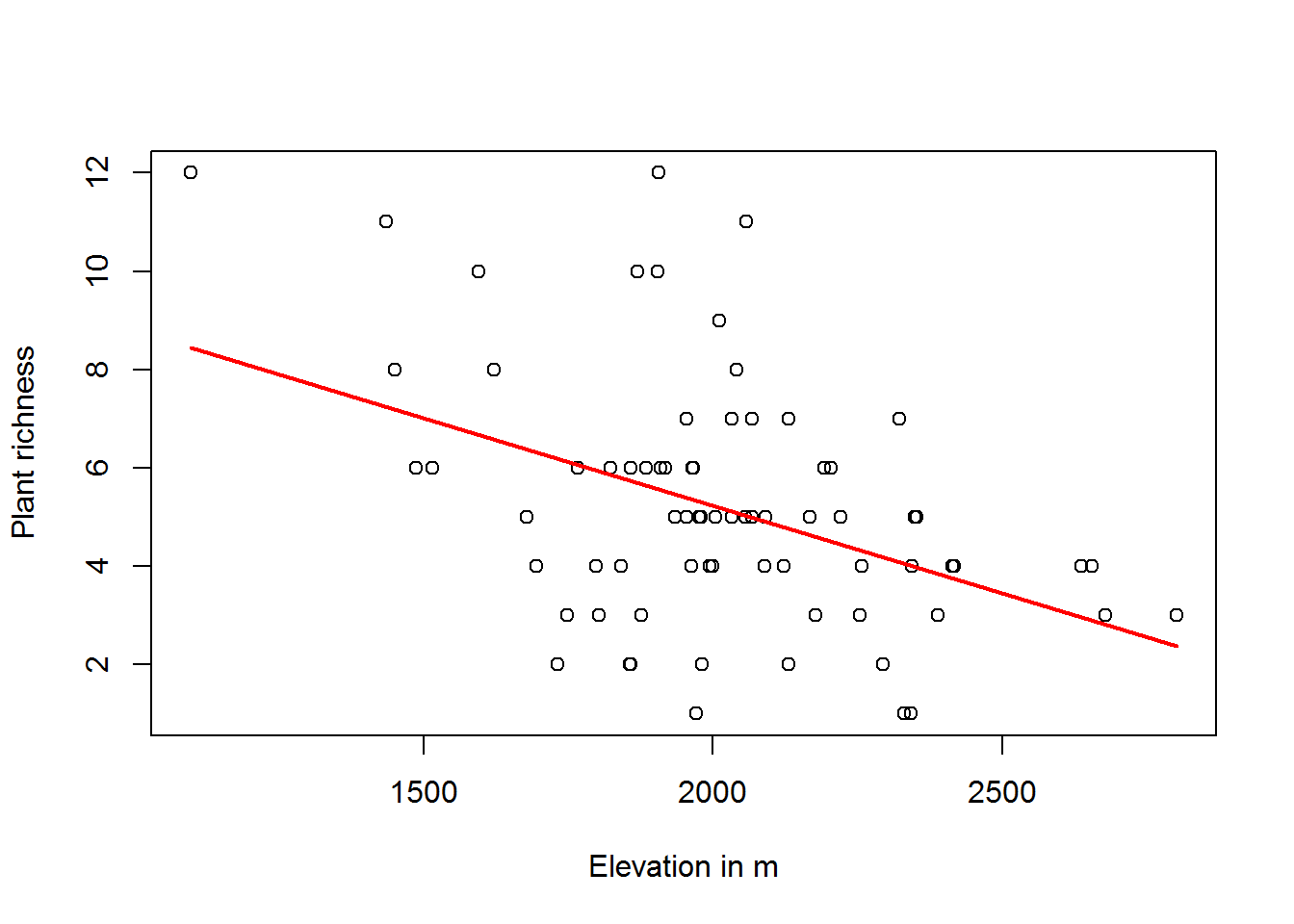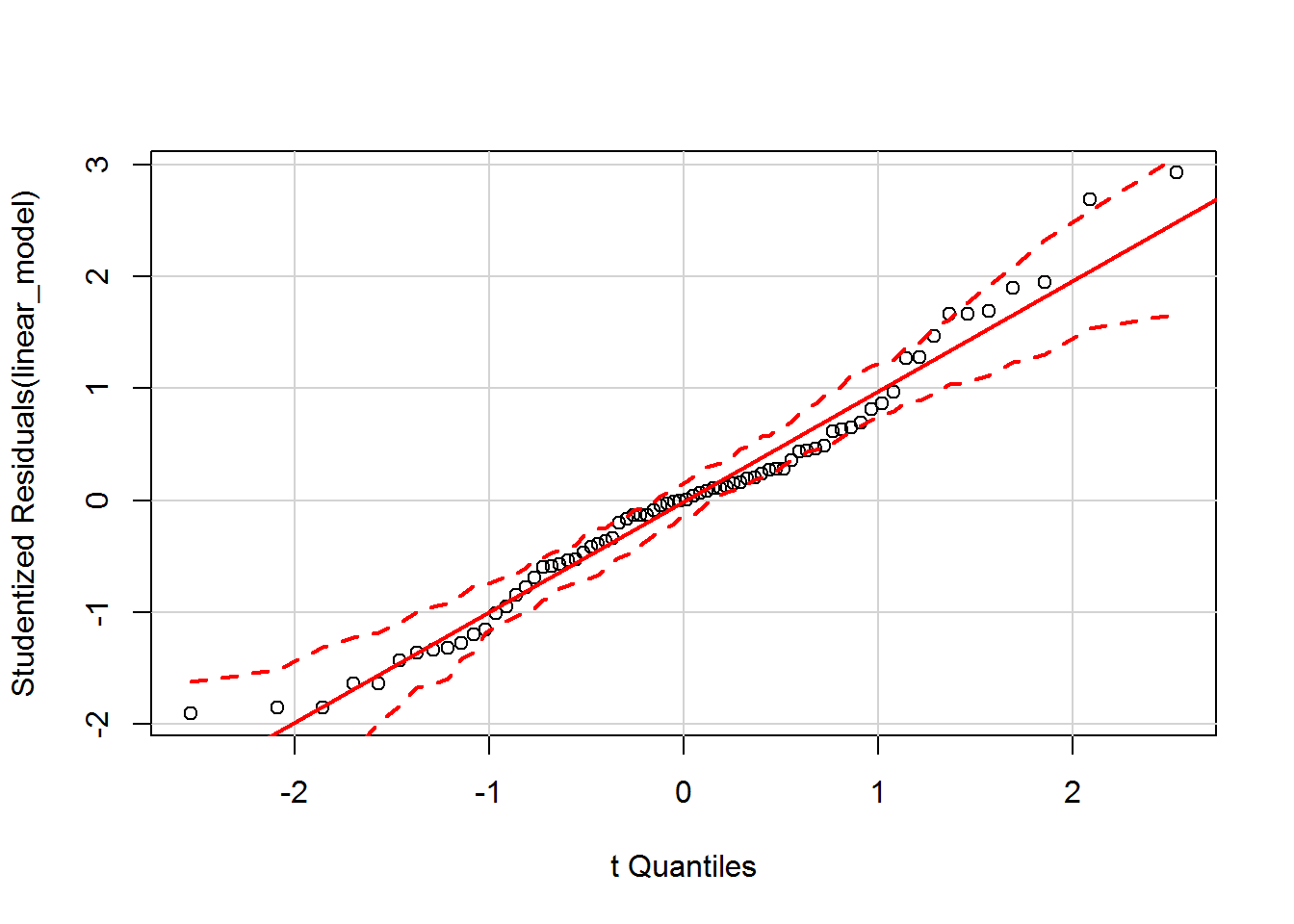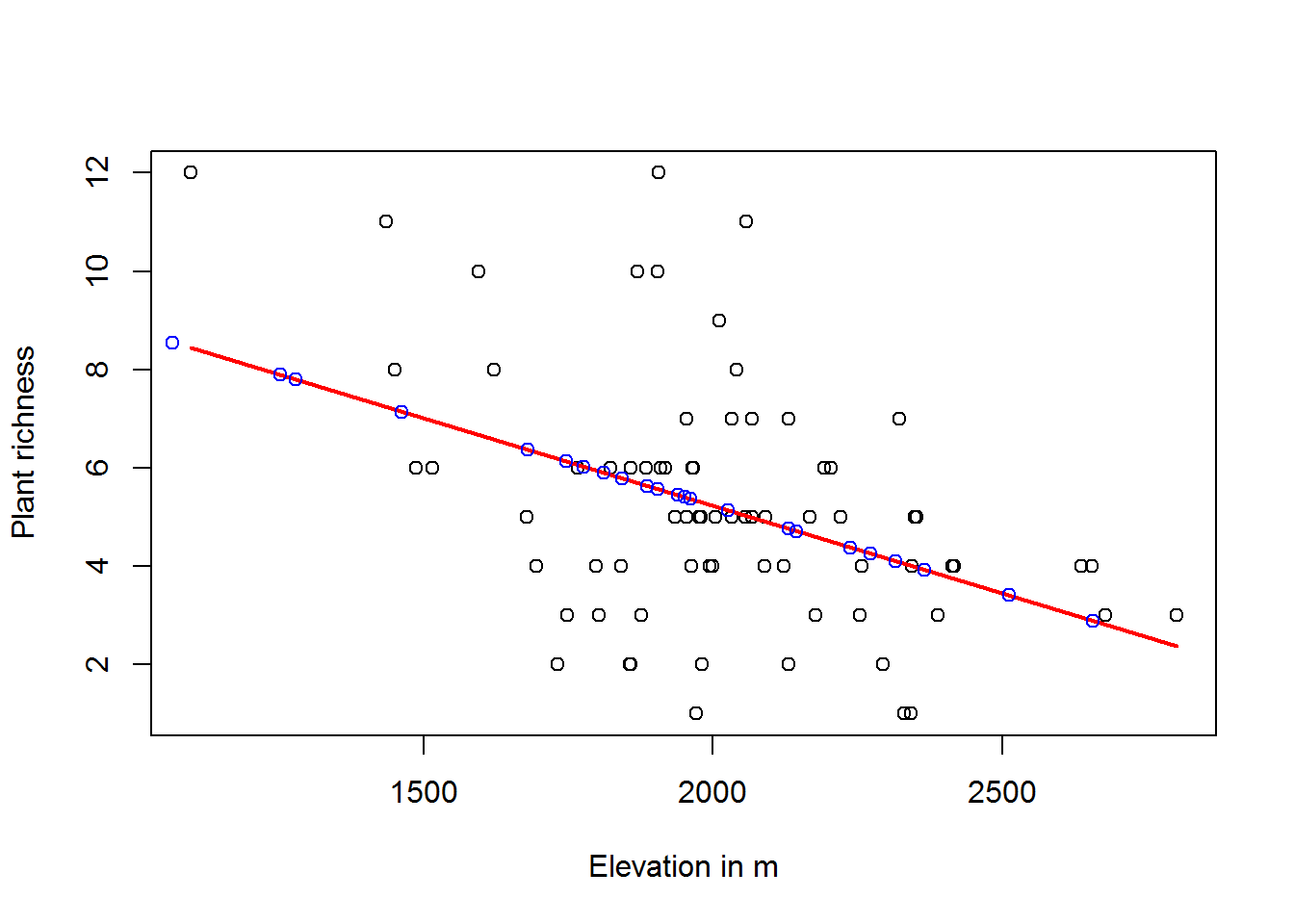#BIS-Fogo

### Site Tools

en:learning:schools:s01:code-examples:ba-ce-05-01

This is an old revision of the document!

### C05-1 Predicting observations with linear models

##### Using linear models for prediction

Once a linear regression model has been derived, it could be used for predicting values of the dependent variable at locations where only the independent variable is avialable (assuming that the model relationship is still valid at these locations).

To illustrate this with the data set at hand, we artificially deleted some of the values of the plant richness and fitted the following linear model to the remaining value pairs of plant richness and elevation.##
## Call:
## lm(formula = richness[train_numbers] ~ data_2007$ALT_GPS_M[train_numbers]) ## ## Residuals: ## Min 1Q Median 3Q Max ## -4.329 -1.348 0.009 1.041 6.439 ## ## Coefficients: ## Estimate Std. Error t value Pr(>|t|) ## (Intercept) 12.354619 1.848619 6.68 4.2e-09 ## data_2007$ALT_GPS_M[train_numbers] -0.003564   0.000908   -3.93    2e-04
##
## (Intercept)                        ***
## data_2007$ALT_GPS_M[train_numbers] *** ## --- ## Signif. codes: 0 '***' 0.001 '**' 0.01 '*' 0.05 '.' 0.1 ' ' 1 ## ## Residual standard error: 2.33 on 72 degrees of freedom ## Multiple R-squared: 0.176, Adjusted R-squared: 0.165 ## F-statistic: 15.4 on 1 and 72 DF, p-value: 0.000196 As one can see, this looks pretty much the same as in the last example where we derived the linear regression model using all available observations. However, if no further validation of the predicted values will be performed, the only estimate of the explanatory power is the r squared value of our linear regression (i.e. roughly 27% explanation). Therefore we have to make sure that at least the residuals of our linear model are normaly distributed: qqPlot(linear_model)Obviously, that is the case (otherwise we could try to solve the problem by transforming the input variables). ##### Computing predictions In order to predict the plant richness for some research plots where we do not have observational plant richness data available, we will apply the linear model equation to these elevation values. Hence, all we need is the intercept and slope value and there we go: intercept <- linear_model$coefficients
slope <- linear_model$coefficients plant_richness_predicted <- slope*elevation_research_plots + intercept To illustrate the result, we add the predicted plant richness to the scatter plot: plot(data_2007$ALT_GPS_M[train_numbers], richness[train_numbers],
xlab="Elevation in m",ylab="Plant richness")
regLine(linear_model)
points(data_2007\$ALT_GPS_M[-train_numbers],plant_richness_predicted,col="blue")Unsurprisingly, they all fall on the regression line (i.e. the linear model).